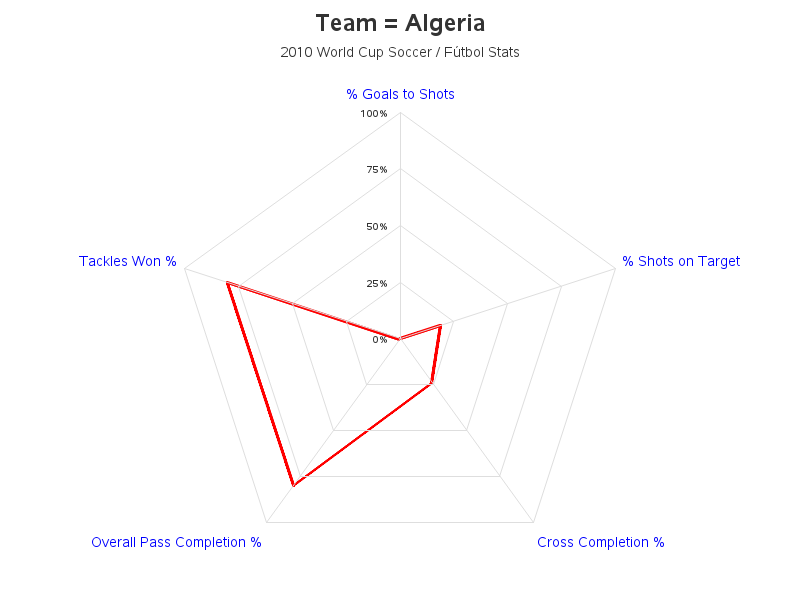## proc gradar - define min and max value and step size for staraxes

I am using this code:

``````AXIS1 Interval=EVEN;

chart Cat1
/
overlay=Cat2
sumvar=Measure
des=''
spiderweb
cstars=(blue, red, black, green)
wstars=4 4 4 4
lstars=1 1 1 1
staraxes= (AXIS1, AXIS1, AXIS1, AXIS1)
;
run;``````

It works OK but is it possible to also define the staraxes to have a min of 0 and max of 10 in step sizes of 0.5? Something along those lines:

``GRID VALUES = (0 TO 10 BY 0.5)``

This:

``AXIS1 Interval=EVEN order=(0 to 10);``

does not product the desired results.

1 ACCEPTED SOLUTION

Accepted Solutions

## Re: proc gradar - define min and max value and step size for staraxes

Here is a gradar chart where I set the axes to go from 0 to 100 by 25. If this is similar to what you're wanting, you should be able to create something similar with your data (the code below should give you some tips):4 REPLIES 4

## Re: proc gradar - define min and max value and step size for staraxes

You have the option Interval=Even on your one shown axis definition. Is your data logarithmic? Note that some of the log related axis statements are noted in the documentation as not supported by GRADAR. Logstyle, Logbase which would be the basic options to control log axis appearance are not supported by GRADAR.

You might try order=(0.5 to 10 by 0.5) without the Interval=Even but I'm not at all sure if that will yield "desired results" with log data. If the data is log then the order values are supposed to match the logbase and logstyle settings, but as documented these aren't supported by Gradar.

## Re: proc gradar - define min and max value and step size for staraxes

Thanks. I tried:

``AXIS1 order=(0.5 to 10 by 0.5)``

Unfortunately this distorts the values, which btw are not logged but simply values between 0 and 10.

## Re: proc gradar - define min and max value and step size for staraxes

Without data is hard to tell what is going "wrong". As a minimum you might post an image of the result you are getting and describe what is undesired or needed.

Best would be to provide some example data in the form of a data step so we can test code.

Why were you using the INTERVAL=Even if the data scale wasn't a log? That is basically the only option involved.

## Re: proc gradar - define min and max value and step size for staraxes

Here is a gradar chart where I set the axes to go from 0 to 100 by 25. If this is similar to what you're wanting, you should be able to create something similar with your data (the code below should give you some tips):